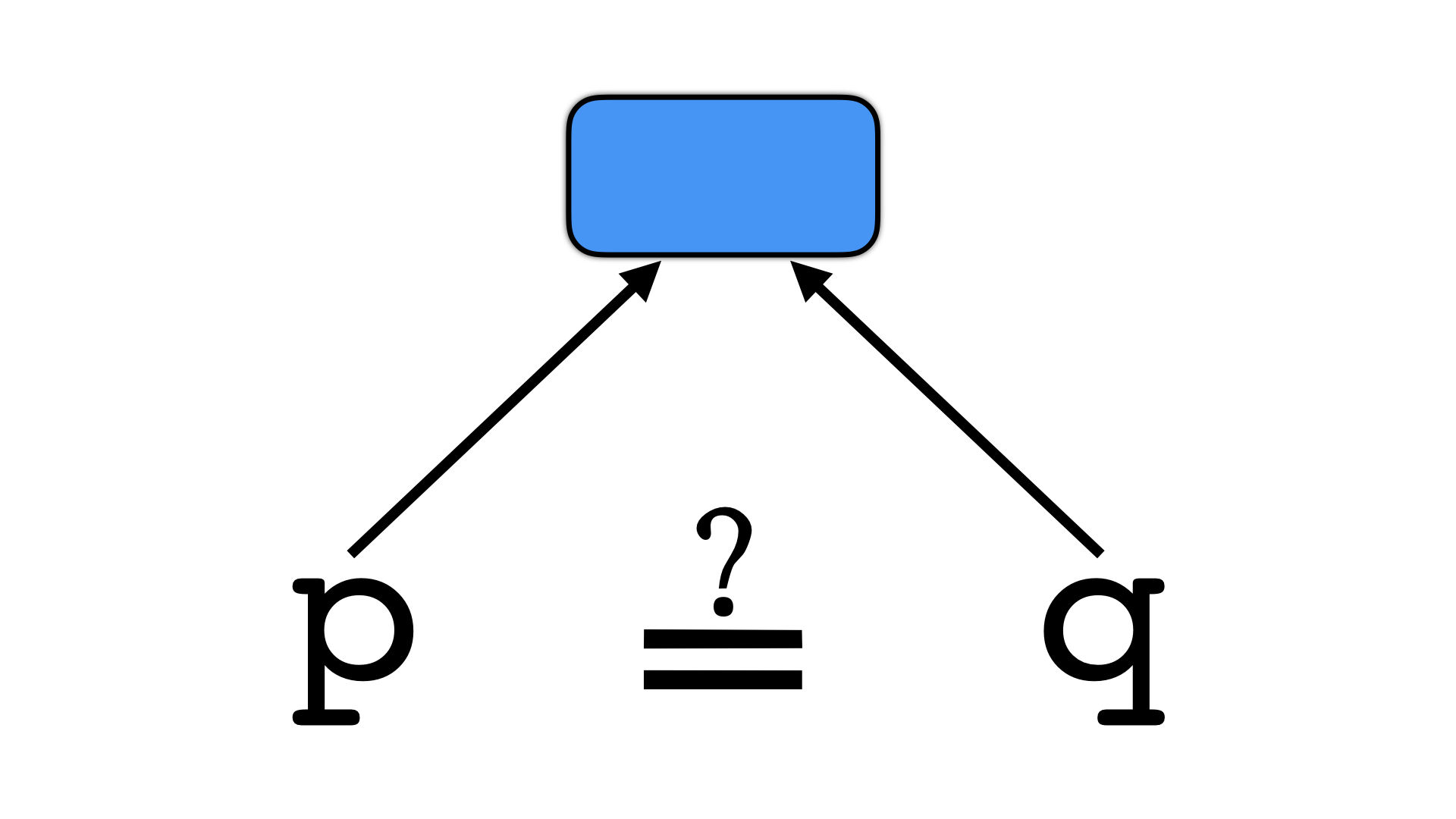# Anti Alias Analysisfree web templates

The goal of this transformation is to disrupt static analysis tools that make use of inter-procedural alias analysis. The current implementation is simplistic: we simply replace all direct function calls with indirect ones.

free web templates

A call to `x = foo(n)` turns into

``````void *arr[]  = {..., & foo, ... };

int main () {
int x = ((int (*)(int n ))arr[expr=42])(n);
}
``````
free web templates

where `arr` is a global array containing function addresses, and `expr=42` is an opaque expression computing the index of `foo`'s address in `arr`. To make analysis a bit harder, we can also insert bogus elements in `arr`, and insert updates to these bogus elements. Here, both `arr` and `arr` are bogus:

``````int main () {
int x;
arr[expr=6] = arr[expr=42];
arr[expr=7] = &x;
}
``````
OptionArgumentsDescription
--Transform AntiAliasAnalysis Transform the code by replacing direct function calls with indirect ones, making alias analysis become less precise.
--AntiAliasAnalysisObfuscateIndex BOOLSPEC Use opaque expressions to compute function addresses. Default=true.
It is best to perform this transformation at the end of the transformation sequence, when all functions (including new ones generated by the virtualize, split, and merge transformations) are known. The reason is that, by definition, this transformation makes alias analysis difficult, which trips up subsequent transformations. This is particularly true for the EncodeData transformation which relies on alias analysis. The `--AntiAliasAnalysisBogusEntries=true` option seems to be particularly troublesome.